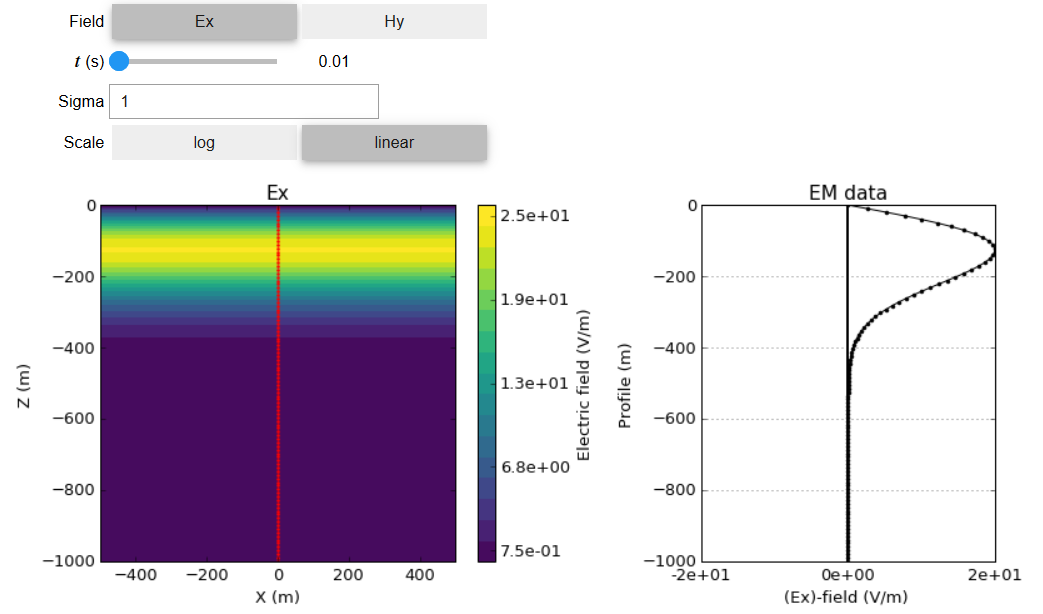# Transient Plane Waves in Homogeneous Media¶

Purpose

We have already shown that in a homogeneous media, electromagnetic signals in the time domain behave according to the wave equation. Here, we discuss the properties of transient planewave solutions in the time domain. Educational content is augmented by using the interactive apps provided to answer a set of fundamental questions. We also discuss the relationships between the electric and magnetic fields carried by planewaves. The content here parallels materials provided in many EM resources (see [WH88][Gri99][Str41]).

Introduction

We have previously shown that in homogeneous media, the propagation of electromagnetic signals in the time domain is governed by the wave equation. One solution to this equation is a planewave, where electric and magnetic fields lie in a 2D plane and the wave propagates in a direction perpendicular to that plane. Physically, the planewave solutions offer good approximations to what is happening far away from electromagnetic sources. As a result, planewaves form a primary basis for understanding the fundamental behaviours of many electromagnetic phenomena.Fig. 51 Geometry of an EM plane wave propagating downwards.

Here, we explore the propagation of planewaves in the time domain (for impulse signals). The content provided here parallels materials from many EM resources (see [WH88][Gri99][Str41]). An app is provided that allow you to explore the concepts of wavelength, attenuation and other aspects of the propagating EM fields. To compliment the app, questions are provided to promote interactive learning. The resource is augmented with derivations and equations that quantify the information learned through using the apps.

Quick Links

Planewave Topics

There are numerous properties which can be used to understand the propagation of planewaves in the time domain. Understanding these properties is very important, as they can be used to describe the behaviours of EM waves in more general cases. Here, we will discuss the following properties:

GeoSci App

While navigating through the subsequent materials on planewaves in homogeneous media, it is suggested that you open the TDEM Planewave Wholespace App from the notebooks page. Don’t forget to sign in.

A fundamental understanding of planewave propagation in the time domain can be obtained by using the TDEM Planewave Wholespace App (Fig. 43); which allows the user to simulate the electric and magnetic fields supported by a downward propagating planewave. The app allows the user to explore the effects of different parameters (e.g. conductivity, observer location, time) and answer a set of fundamental questions. For example, assume that an impulse excitation sends an EM planewave signal into the Earth and that the ground has a conductivity of 1 S/m.

• What is the peak amplitude (maximum amplitude) at $$t$$ = 0.01 s?

• At what time does the peak amplitude of the wave cross 600 m depth?

A host of additional questions which can be answered using the app are found here.Fig. 52 Screen shot of the TDEM planewaves wholespace app.

Contents